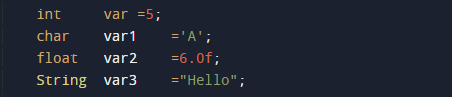# Variables Initialization Program in Java

In this tutorial you will learn about the Variables Initialization Program in Java and its application with practical example.

In this tutorial, we will learn to create a Java to Program Variables Initialization Program in Java using java programming.

## Prerequisites

Before starting this tutorial, we assume that you are the best aware of the following Java programming concepts.

• Java Operators.
• Basic Input and Output function in Java.
• Class and Object in Java.
• Basic Java programming.

## Variables Initialization in Java

Just like other programming languages, we can declare and initiate variables in Java, here is the syntax and example of how we can declare a variable and initiate values of a variable.

“Data_Type => variable_name”
variable_name=value;
int a= 10;

## Variables Initialization Program in Java

In this program, we will find the smallest element in an array using a nested for loop. We would first declare and initialized the required variables. Next, we will find the smallest number in an array. Let’s have a look at the code.

## Output

Printing the values of variablesIn the above program, we have first declared and initialized a set of variables required in the program.

• var    = it will hold integer value.
• var1 = it will hold character value.
• var2 = it will hold float value.
• var3 = it will hold string value.

After declaring variables we initiate values in variables.Now we will print the values of the variables.## Declaration:=>

The process of Declaration is by defining the variable along with its type and name.
Example:=> we are declaring a variable named var variable.
int var;

## Initialization:=>

here we assign a value to a variable as shown below
Example: Var = 10;

In this tutorial we have learn about the Variables Initialization Program in Java and its application with practical example. I hope you will like this tutorial.